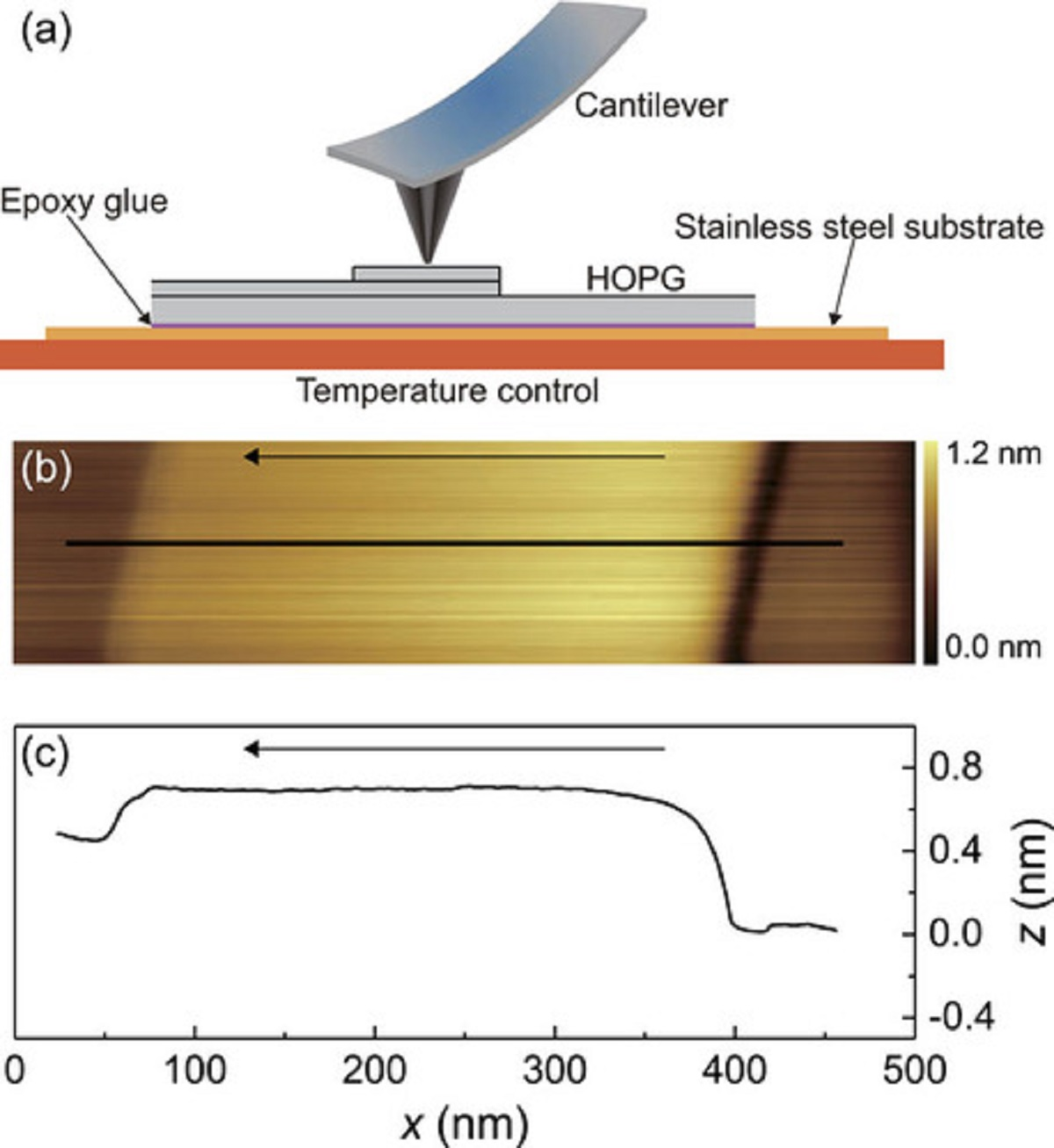# Temperature effects on the nano-friction across exposed atomic step edges

In the article “Temperature effects on the nano-friction across exposed atomic step edges” Wen Wang, Ashu Wang and Lingyan Zeng describe how they used friction force microscopy ( FFM ) under ultrahigh vacuum ( UHV) conditions to study the temperature dependence of nanoscale friction between a silicon AFM tip ( NANOSENSORS™ PointProbe® Plus PPP-LFMR AFM probe for lateral/friction force microscopy ) and a freshly cleaved HOPG surface with exposed single- and double-layer step edges.*

They present experimental measurements as well as theoretical calculations of the temperature effects on atomic friction across HOPG surface step edges.*

Among other things the authors found that the resistive force for the double-layer step edge was twice as large as that of the single-step edge, and simultaneously, the assistive force that resulted from the horizontal component of the total force acting on the AFM tip seemed to be less influenced by the height of the step edges.*

Their experimental results also showed that temperature had very little effect on the friction coefficients at the step edges, which is inconsistent with the thermal activated friction where friction should decrease with temperature.*

Based on the theoretical studies, this observation can be explained by a process where the temperature effect is very small compared with the edge Schwoebel–Ehrlich barrier.*

The authors hope that their findings will contribute to understanding the temperature effects on macroscopic friction having a lot of step edges at the interface.*Figure 1 from “Temperature effects on the nano-friction across exposed atomic step edges” by Wen Wang et al.: Experimental setup and the topographic image of the HOPG surface with step edges used in our measurements. (a) Illustration of the experimental setup. All experiments have been performed using a conventional friction force microscope on a freshly cleaved HOPG sample which was in contact with the temperature control stage under UHV conditions. (b) The typical topographic image of the HOPG surface with a single- and double-layer step edge obtained at T = 297.7 K using the contact mode operation with an applied normal force of 13.1 nN and a scan velocity of 1.25 μm/s. (c) The cross-section height profile across the step edges highlighted in (b). The black arrows in (b) and (c) indicate the scanning direction.

*Wen Wang, Ashu Wang and Lingyan Zeng
Temperature effects on the nano-friction across exposed atomic step edges